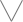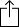C
D
E
F
G
A
B
Chord
Scale
3nps
CAGED
None
Notes
Degrees
Intervals
None

1
2
3
4
5
1
2
3
4
5
6
7
8
9
10
11
12
13
14
15
16
17
18
19
20
21
22
23
24
E
B
G
D
A
E
B
F
F
G
A
A
B
B
C
D
D
E
E
F
F
G
A
A
B
B
C
D
D
E
E
C
D
D
E
E
F
F
G
A
A
B
B
C
D
D
E
E
F
F
G
A
A
B
B
A
A
B
B
C
D
D
E
E
F
F
G
A
A
B
B
C
D
D
E
E
F
F
G
E
E
F
F
G
A
A
B
B
C
D
D
E
E
F
F
G
A
A
B
B
C
D
D
B
B
C
D
D
E
E
F
F
G
A
A
B
B
C
D
D
E
E
F
F
G
A
A
F
F
G
A
A
B
B
C
D
D
E
E
F
F
G
A
A
B
B
C
D
D
E
E
C
D
D
E
E
F
F
G
A
A
B
B
C
D
D
E
E
F
F
G
A
A
B
B

# E Half Diminished for 7 String Guitar - CAGED Chart

E Half diminished, sometimes called "E Locrian #2" is a diatonic scale and is the 6th mode in the set of Melodic minor modes

E
F
G
A
B
C
D
EW
H
W
H
W
W
W
Play scale
Install fretastic on your iPhone:
tapand then
"Add to homescreen"